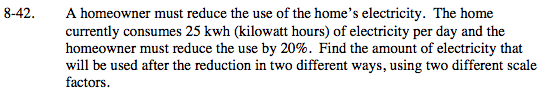### Home > MC2 > Chapter 8 > Lesson 8.2.2 > Problem8-42

8-42.Find 20% of the amount of electricity used.

$\frac{20}{100} \left(\frac{?}{?}\right) = \frac{x}{25}$

Once you have found the amount of reduction, subtract that from the original amount of power consumption.

25 − 5 = 20 kwh
If you reduce the use of electricity by 20%, what percentage is left?